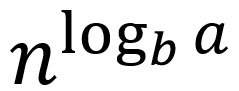# 雅乐网

## 使用主定理求解递归式

### 主定理的三种情况#### 情况二：

f(n)和同阶，这时候T(n) = θ(*  log n ) =  θ( f(n)  *  log n )

#### 情况三：

f(n)多项式渐进大于,  T(n) =θ ( f(n) )

### 例子#### T(n) = T(2n/3) + 1

a = 1, b = 3/2 , f(n) = 1= n^0 = 1 ,和1是同阶的，适应情况二。

T(n) = O(* logn) = θ(logn)

#### T(n) = 3T(n/4) + nlogn

a = 3, b = 4, f(n) = n*logn.

log(3,4)约等于0.793= n^0.793，虽然不准确，但是肯定小于n^1，和f(n)=nlogn进行比较，虽然f(n)包含logn的形式，但只看n的部分也至少要少了n^0.2 ，这个也是多项式意义上的，适用情况三。

3* n/4 log(n/4) < c n logn

T(n) = θ (nlogn)

#### T(n)=2T(n/2)+nlogn

a = 2, b = 2, f(n) = nlogn= n 。我们注意到n确实是小于nlogn的，但是这并不是多项式意义上的小于，因为两个式子n的次数都是1，因此不能应用主定理。

http://www.yalewoo.com/master_theorem.html